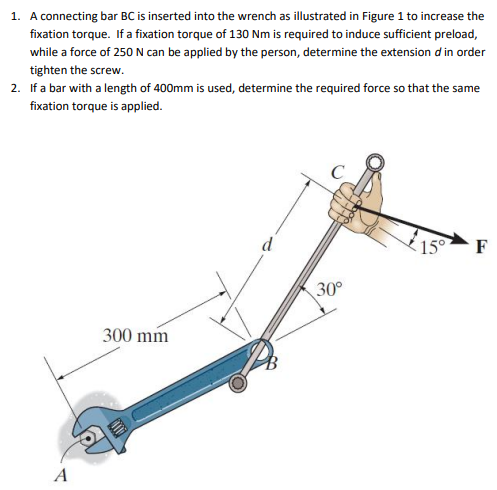Home / Expert Answers / Physics / 1-a-connecting-bar-bc-is-inserted-into-the-wrench-as-illustrated-in-figure-1-to-increase-the-fixa-pa263

# (Solved): 1. A connecting bar BC is inserted into the wrench as illustrated in Figure 1 to increase the fixa ...1. A connecting bar BC is inserted into the wrench as illustrated in Figure 1 to increase the fixation torque. If a fixation torque of $$130 \mathrm{Nm}$$ is required to induce sufficient preload, while a force of $$250 \mathrm{~N}$$ can be applied by the person, determine the extension $$d$$ in order tighten the screw. 2. If a bar with a length of $$400 \mathrm{~mm}$$ is used, determine the required force so that the same fixation torque is applied.

We have an Answer from Expert